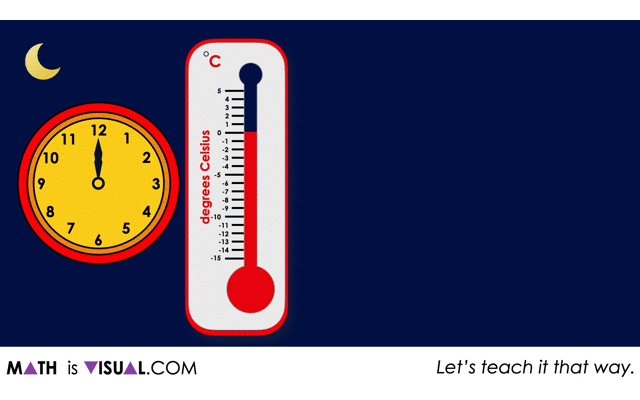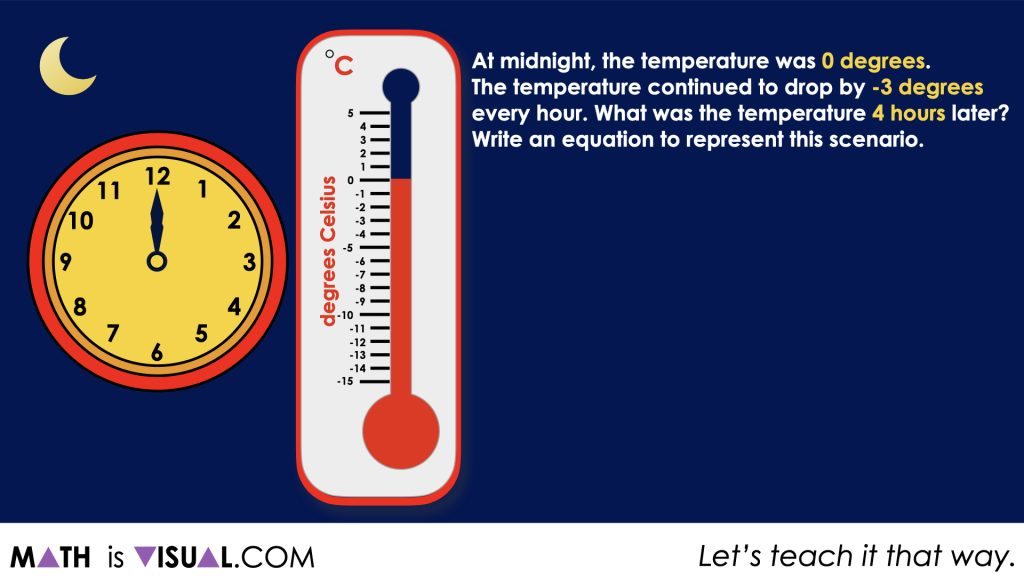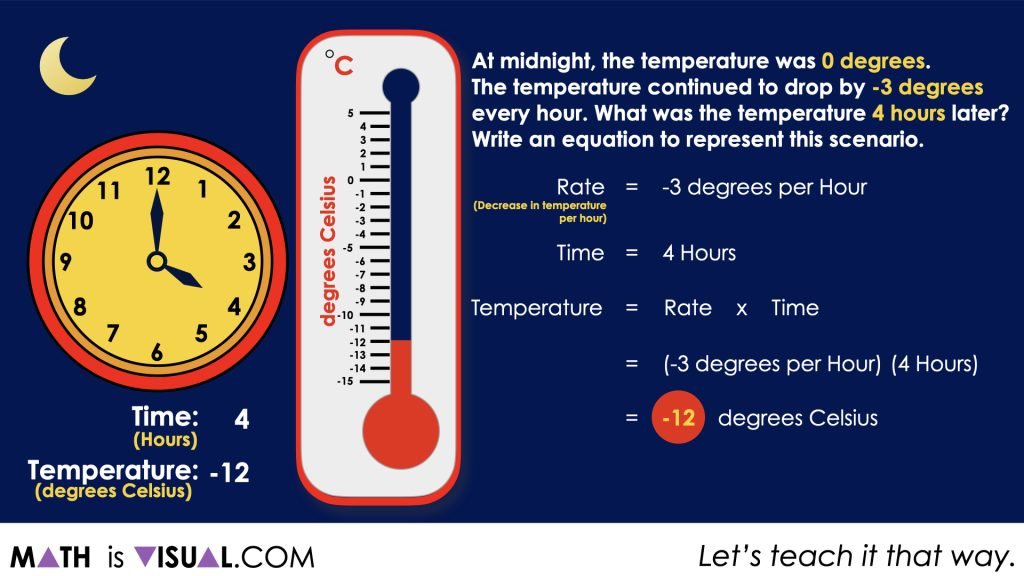# Real World Integer MultiplicationExplore a real world integer multiplication context involving temperature where the rate is negative (decreasing hourly temperature) over time.

https://youtu.be/zyCoyIcTPrU

## In This Set of Visual Number Talk Prompts…

Through this visual math talk and purposeful practice, students are encouraged to use the number line to model the behaviour of multiplying and dividing positive and negative integers. All division scenarios today will be quotative.

## Intentionality…

The purpose of the Day 2 activities is to reinforce key concepts from Day 1. Students will also be introduced to a new multiplication scenario where a negative integer is multiplied by a negative integer. Students will engage in a math talk and will have an opportunity to complete independent purposeful practice. The math talk and purposeful practice serve to develop a deeper understanding of the following big ideas.

• Multiplication can be understood as repeated equal groups;
• The first factor can be understood as the number of groups and the second factor as the size of the groups;
• A positive integer multiplied by a negative integer has a product that is negative;
• A negative integer multiplied by a negative integer has a product that is positive;
• Quotative division is when the total quota is known (the dividend), and the number per group or the rate (the divisor) is known;
• Quotative division reveals the number of copies or iterations of a rate that can be derived from the overall quota (the dividend);
• A negative number divided by a negative number has a quotient that is positive.

## Visual Math Talk Prompt #1

Students will be prompted with:

At midnight, the temperature was 0 degrees.

The temperature continued to change by -3 degrees every hour.

What was the temperature 4 hours later?

Write an equation to represent this scenario.From the animation, students will see that with every passing hour, the temperature drops by 3 degrees (or the change in temperature is -3 degrees per hour).

With this, students might create a table to track the number of hours and the current temperature beginning at 0 hours (12:00 am) and 0 degrees, then 1 hour (1:00 am) and -3 degrees, and so on.

Some students may recognize that they can simply multiply the rate of -3 degrees per hour by 4 hours giving a result of -12 degrees Celsius after the 4th hour.Facilitator note:

The purpose of this first scenario is to solidify the following big ideas.

• Multiplication can be understood as repeated equal groups;
• The first factor can be understood as the number of groups and the second factor as the size of the groups;
• A positive integer multiplied by a negative integer has a product that is negative.

## Want to Explore These Concepts & Skills Further?

Two (2) additional number talk prompts are available in Day 2 of the Just Keep Digging problem based math unit that you can dive into now.

Why not start from the beginning of this contextual 5-day unit of real world lessons from the Make Math Moments Problem Based Units page.

Did you use this in your classroom or at home? How’d it go? Post in the comments!

Math IS Visual. Let’s teach it that way.